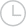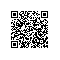# 如何在keras中对张量进行排序.-问答-阿里云开发者社区-阿里云

## 如何在keras中对张量进行排序.邻泽居士2019-04-25 10:29:102396取消 提交回答

• 张维ACE
2019-09-10 20:46:46
推荐
``````import numpy as np

a = np.array([[1,2],[3,4]])
sum0 = np.sum(a, axis=0)
sum1 = np.sum(a, axis=1)

print (sum0)
print (sum1)
``````

输出的结果为：[4,6]                                      [3,7]          在计算的时候，当[Math Processing Error]axis=0的时候是矩阵的列向量相加，当[Math Processing Error]axis=1的时候是矩阵的行向量相加。 ————

0 0使用钉钉扫一扫加入圈子
+ 订阅